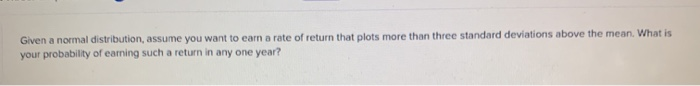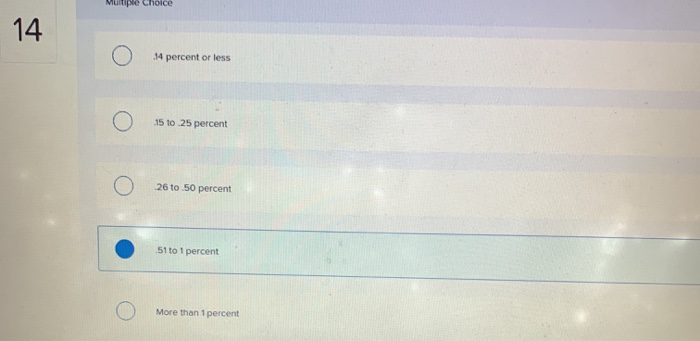### Create an Account

Home / Questions / Given a normal distribution, assume you want to earn a rate of r

# Given a normal distribution, assume you want to earn a rate of rGiven a normal distribution, assume you want to earn a rate of return that plots more than three standard deviations above the mean. What is your probability of eaming such a return in any one year?
Multiple Choice o 4 percent or less o О 15 to 25 реrсеnt o 26 tо 50 percent o 51 to 1 percent o More than 1 percent

Aug 19 2020 View more View LessSubscribe To Get Solution